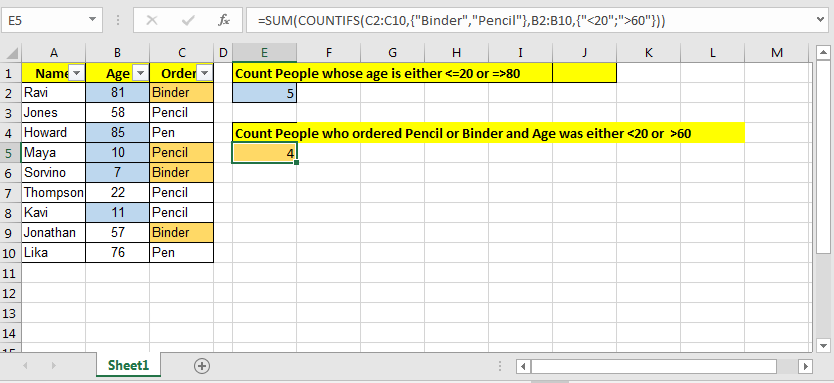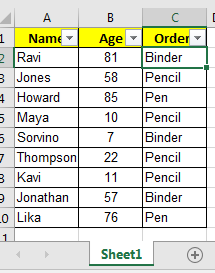# How to COUNTIFS With OR For Multiple Criteria in Excel

Basically, when we supply multiple conditions to a COUNTIFS function, it checks all the conditions and returns row which matches all the conditions. Which means it runs on AND logic.But what if you want to count on an OR logic? We need to accomplish COUNTIFS with OR logic.
This can be done easily with arrays and SUM function. Let's see how…
Generic Formula To Countif With OR Logic For Multiple Optional Conditions

=SUM(COUNTIFS(range{condition1, condition2,...})

Example COUNTIFS with OR
So this one time, I had this data. Where I needed to count people who had aged less than or equal to 20 OR greater than or equal to 80. I did it using SUM function and arrays. Come, I’ll show you how.So I had these two conditions (“<=20”,”>=80”). Either of them needed to be true to be counted. So I wrote this COUNTIF formula

=SUM(COUNTIFS(B2:B10,{"<=20",">=80"})

It gave the correct answer as 5.

How does it work?
The COUNTIFS matched each element of array {"<=20",">=80"} and gave count of each item in the array as {3,2}.
Now you know how SUM function in Excel works. SUM function added the values in the array(SUM({3,2}) and gives us the result 5. This crit

Let me show you another example of COUNTIFS with multiple criteria with or logic. This time I will not explain it to you. You need to figure it out.

COUNTIFS Multiple Criteria Example Part 2
Now this one is the final one. Here I had two sets of OR conditions and one AND. So the query was:
Count the people who ordered Pencil OR Binder AND Age was either 60:
It was tricky but i figured it out. Here is the formula.

=SUM(COUNTIFS(C2:C10,{"Binder","Pencil"},B2:B10,{"<20";">60"}))

This gave me the exact count of 4 for the query.

How It Worked
Actually here I used two-dimensional arrays. Note that semicolon in {"<20";">60"}. It adds a second dimension or says columns to the array as {1,2;1,0}.
So basically, first it finds all the binders or pencils in range C2:C10. Then in the found list, it checks who are under the age of 20 or more aged than 60.

So what you think. I used COUNTIFS to count multiple criteria with multiple conditions. First I told how I used excel countifs two criteria match and then we used countifs multiple criteria match with or logic. Don’t worry about the version of excel. It will work in Excel 2016, Excel 2013, Excel 2010 and older which have the COUNTIF function and concept of array formulas.

Related Articles:

How to COUNTIFS Two Criteria Match in Excel

How to Count Cells That Contain This Or That in Excel

How To Count Unique Text in Excel

Popular Articles:

50 Excel Shortcuts to Increase Your Productivity

How to use the VLOOKUP Function in Excel

How to use the COUNTIF function in Excel

How to use the SUMIF Function in Excel

1.Robert McGechan

I have a spreadsheet where I believe I use CountIfs formula:
=CountIfs(Range,"1=1")
Is this a correct formula?
If I want all the returned values to be added together eg: 1+0+1+1=3 etc should I use SumCountIfs?

•it should be =COUNTIF(RANGE,1)

It will return the total count of 1's in the range. You may not need to use sumif function.

Terms and Conditions of use

The applications/code on this site are distributed as is and without warranties or liability. In no event shall the owner of the copyrights, or the authors of the applications/code be liable for any loss of profit, any problems or any damage resulting from the use or evaluation of the applications/code.